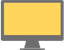Some test text!

# Search PDF files for text in Obj-C

More languages

chevron_right
More languages
JavaScript
Java (Android)
C++
C#
C# (.NET Core)
Java
Kotlin
Obj-C
JS (Node.js)
PHP
Python
Ruby
Swift
C# (UWP)
VB
C# (Xamarin)

Sample Obj-C code for using PDFTron SDK to search text on PDF pages using regular expressions. The TextSearch utility class builds on functionality available in TextExtractor to simplify most common search operations. Learn more about our Obj-C PDF Library and PDF Indexed Search Library.

To run this sample, get started with a free trial of PDFTron SDK.

``````//---------------------------------------------------------------------------------------
// Consult legal.txt regarding legal and license information.
//---------------------------------------------------------------------------------------

#import <OBJC/PDFNetOBJC.h>
#import <Foundation/Foundation.h>

// This sample illustrates the basic text search capabilities of PDFNet.

int main(int argc, char *argv[])
{
@autoreleasepool {

int ret = 0;
[PTPDFNet Initialize: 0];
NSString *input_path = @"../../TestFiles/credit card numbers.pdf";

@try
{
PTPDFDoc *doc = [[PTPDFDoc alloc] initWithFilepath: input_path];
[doc InitSecurityHandler];

PTTextSearch *txt_search = [[PTTextSearch alloc] init];
unsigned int mode = e_ptwhole_word | e_ptpage_stop;
NSString *pattern = @"joHn sMiTh";

//call Begin() method to initialize the text search.
[txt_search Begin: doc pattern: pattern mode: mode start_page: -1 end_page: -1];

int step = 0;

//call Run() method iteratively to find all matching instances.
while ( YES )
{
PTSearchResult *result = [txt_search Run];

if ( result )
{
if ( step == 0 )
{	//step 0: found "John Smith"
//note that, here, 'ambient_string' and 'hlts' are not written to,
//as 'e_ambient_string' and 'e_highlight' are not set.

NSLog(@"%@'s credit card number is: ", [result GetMatch]);
//now switch to using regular expressions to find John's credit card number
mode = [txt_search GetMode];
mode |= e_ptreg_expression | e_pthighlight;
[txt_search SetMode: mode];
pattern = @"\\d{4}-\\d{4}-\\d{4}-\\d{4}"; //or "(\\d{4}-){3}\\d{4}"
[txt_search SetPattern: pattern];

++step;
}
else if ( step == 1 )
{
//step 1: found John's credit card number
NSLog(@"  %@", [result GetMatch]);

//note that, here, 'hlts' is written to, as 'e_highlight' has been set.
//output the highlight info of the credit card number.
PTHighlights *hlts = [result GetHighlights];
[hlts Begin: doc];
while ( [hlts HasNext] )
{
NSLog(@"The current highlight is from page: %d", [hlts GetCurrentPageNumber]);
[hlts Next];
}

//see if there is an AMEX card number
pattern = @"\\d{4}-\\d{6}-\\d{5}";
[txt_search SetPattern: pattern];

++step;
}
else if ( step == 2 )
{
//found an AMEX card number
NSLog(@"\nThere is an AMEX card number:\n  %@", [result GetMatch]);

//change mode to find the owner of the credit card; supposedly, the owner's
//name proceeds the number
mode = [txt_search GetMode];
mode |= e_ptsearch_up;
[txt_search SetMode: mode];
pattern = @"[A-z]++ [A-z]++";
[txt_search SetPattern: pattern];

++step;
}
else if ( step == 3 )
{
//found the owner's name of the AMEX card
NSLog(@"Is the owner's name:\n  %@?\n", [result GetMatch]);

//add a link annotation based on the location of the found instance
PTHighlights *hlts = [result GetHighlights];
[hlts Begin: doc];
while ( [hlts HasNext] )
{
PTPage *cur_page = [doc GetPage: [hlts GetCurrentPageNumber]];
int i = 0;
for ( ; i < [quads size]; ++i )
{
//assume each quad is an axis-aligned rectangle
double x1 = MIN(MIN(MIN([[q getP1] getX], [[q getP2] getX]), [[q getP3] getX]), [[q getP4] getX]);
double x2 = MAX(MAX(MAX([[q getP1] getX], [[q getP2] getX]), [[q getP3] getX]), [[q getP4] getX]);
double y1 = MIN(MIN(MIN([[q getP1] getY], [[q getP2] getY]), [[q getP3] getY]), [[q getP4] getY]);
double y2 = MAX(MAX(MAX([[q getP1] getY], [[q getP2] getY]), [[q getP3] getY]), [[q getP4] getY]);
PTPDFRect * rect = [[PTPDFRect alloc] initWithX1: x1 y1: y1 x2: x2 y2: y2];
PTAction *action = [PTAction CreateURI: [doc GetSDFDoc] uri: @"http://www.pdftron.com"];

}
[hlts Next];
}
[doc SaveToFile: @"../../TestFiles/Output/credit card numbers_linked.pdf" flags: e_ptlinearized];

break;
}
}

else if ( [result IsPageEnd] )
{
//you can update your UI here, if needed
}

else
{
break;
}
}
}

@catch(NSException *e)
{
NSLog(@"%@", e.reason);
ret = 1;
}
return ret;
}
}``````
close

## Free Trial

Get unlimited trial usage of PDFTron SDK to bring accurate, reliable, and fast document processing capabilities to any application or workflow.

Select a platform to get started with your free trial.

Unlimited usage. No email address required.